#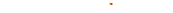###### Scale Texture 1 and Texture 2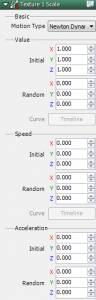Basics Motion Type
 Constant Keep size fixed Newton Dynamics Set size based on values speed and acceleration Curve Value Set fixed size for keyframes in Timeline Curve Speed Set scale speed for keyframes in Timeline

Constant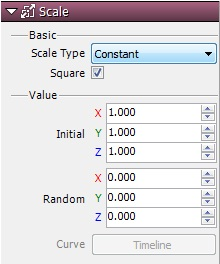Set the initial size and from there select do not move. Compared to other types of functions, one of the characteristics of “Constant” is the processing load on the CPU is light.

Newton Dynamics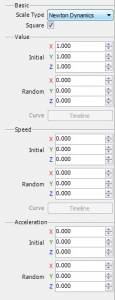In addition to values, set Move speed and acceleration.
 Value Specify the initial size of texture. The random value is the initial scale with a random range. The random range is between – (specified value) and + (specified value). Speed Specify the scale speed of texture. The random value is the speed with a random range. The random range is between – (specified value) and + (specified value). Acceleration Specify the scale acceleration of texture. The random value is the acceleration with a random range. The random range is between – (specified value) and + (specified value).

Curve Value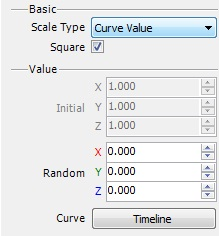Set scale values for graphs in Timeline. Change keyframes in real time as keyframes run.

Curve Speed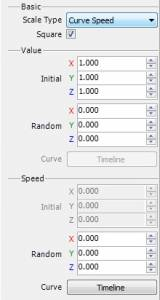Set speed values for graphs in Timeline. Change keyframes in real time as keyframes run.